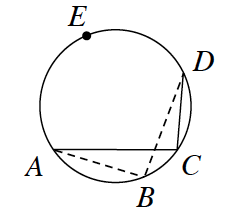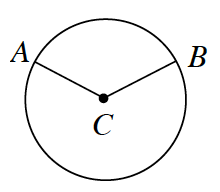### Home > INT2 > Chapter 10 > Lesson 10.2.4 > Problem10-97

10-97.

Review what you know about the angles and arcs of circles below.

1. A circle is divided into nine congruent sectors. What is the measure of each central angle?

Recall that a whole circle is $360°$.

2. In the diagram at right, what are $m\overarc{ AED }$ and $m∠C$ if $m∠B = 97°$?

Refer to the Math Notes in this lesson.

Notice the relationship between $m∠B$ and $m∠C$.3. In $⊙C$ at right, $m∠ACB = 125°$ and $BC = 8$ units. What are $m\overarc { A B }$ and the length of $\overarc { A B }$? What is the area of the smaller sector?

What portion of the circumference is $\overarc { A B }$?

Use the same method as above to find the area of the smallest sector.